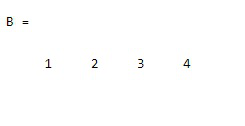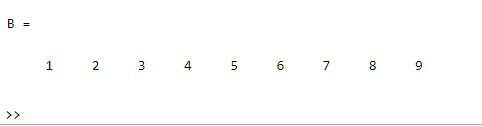Related Articles

# Turn a Matrix into a Row Vector in MATLAB

• Last Updated : 04 Jul, 2021

Conversion of a Matrix into a Row Vector. This conversion can be done using reshape() function along with the Transpose operation. This reshape() function is used to reshape the specified matrix using the given size vector.

### Syntax:

`reshape(A, sz)`

Parameters: This function accepts two parameters, which are illustrated below:

• A: This is the specified matrix of elements.
• sz: This is the specified size vector.

Return Value: It returns the row vector of a given matrix.

Example 1

## Matlab

 `% Conversion of a 2D matrix into its``% row vector.``A = [1 2; 3 4]; ``%Initializing a matrix`` ` `% Calling the reshape() function``% over the above matrix as its transpose``% and size vector as 1,[]``B = reshape(A.',1,[])`

Output:Example 2

## Matlab

 `%  MATLAB code for Conversion of a 3*3``% matrix into its row vector.``A = [1 2 3; 4 5 6; 7 8 9]; ``% Initializing a 3*3 matrix`` ` `% Calling the reshape() function``% over the above matrix as its transpose``% and size vector as 1,[]``B = reshape(A.',1,[])`

Output:My Personal Notes arrow_drop_up